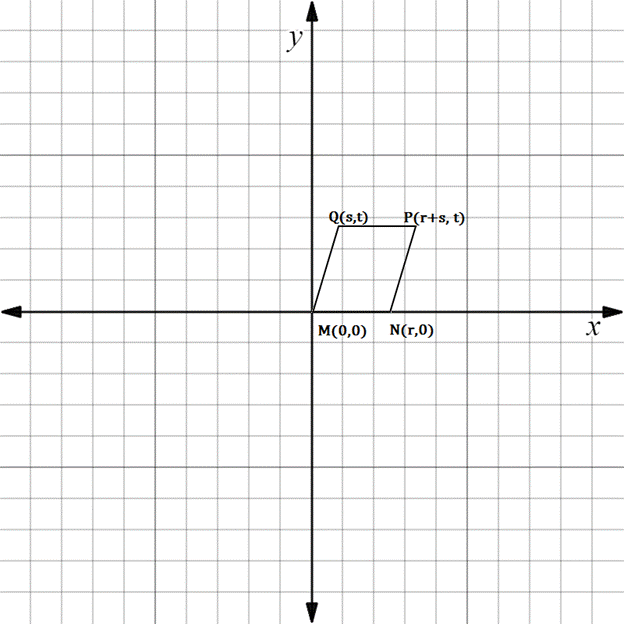Chapter 10.3, Problem 23EElementary Geometry For College St...

7th Edition
Alexander + 2 others
ISBN: 9781337614085

Solutions

Chapter
SectionElementary Geometry For College St...

7th Edition
Alexander + 2 others
ISBN: 9781337614085
Textbook Problem

In Exercises 23 to 28, find the equation (realtionship) requested. Then eliminate fractions and square root radicals from the equation.If ▱ M N P Q is a rhombus, state an equation that relates r , s , and t .To determine

To state:

An equation that relates r,s, and t for the given rhombus.

Explanation

The given figure is shown below.

If the given figure represents a rhombus, it is necessary that MN=MQ.

First find the distance MN.

From the figure it is observed that the distance MN=r.

To find the distance MQ, we have to use the distance formula.

x1,y1=M0, 0

x2,y2=Qs, t

Formula for distance between two points

d=x2-x12+y2-y12

Substituting the x and y co-ordinates

dMQ=s-02+

Still sussing out bartleby?

Check out a sample textbook solution.

See a sample solution

The Solution to Your Study Problems

Bartleby provides explanations to thousands of textbook problems written by our experts, many with advanced degrees!

Get Started

23. For the cost function , show that average costs are minimized at the x-value where

Mathematical Applications for the Management, Life, and Social Sciences

Differentiate. y=tsint1+t

Calculus (MindTap Course List)

Define the terms population and sample, and explain the role of each in a research study.

Essentials of Statistics for The Behavioral Sciences (MindTap Course List)

In Exercises 107-120, factor each expression completely. 117. 9x2 16y2

Applied Calculus for the Managerial, Life, and Social Sciences: A Brief Approach